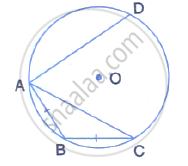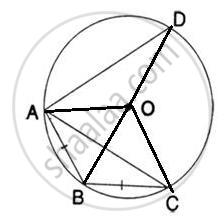Share

# In the Given Diagram, Chord Ab = Chord Bc.I) What is the Relation Between Arcs Ab and Bc? (Ii) What is the Relation Between ∠Aob and ∠Boc?If Arc Ad is Greater than Arc Abc, Then What is the Relation - Mathematics

Course

#### Question

In the given diagram, chord AB = chord BC.(i) what is the relation between arcs AB and BC?
(ii) what is the relation between ∠AOB and ∠BOC?
(iii) If arc AD is greater than arc ABC, then what is the relation between      chords AD and AC?
(iv) If ∠AOB = 50°, find the measure of angle BAC.

#### Solution

Join OA, OB, OC and OD.(i) Arc AB = Arc BC      [ ∵ Equal chords subtends equal arcs]

(ii) ∠AOB = ∠BOC       [ ∵ Equal chords subtends equal arcs]

(iii) If arc AD > arc ABC, then chord AD > AC

(iv) ∠AOB = 50°

But ∠AOB = ∠BOC      [from (ii) above]
∴  ∠BOC = 50°

Now arc BC subtends ∠BOC at the center and ∠BAC at The remaining part of the circle.
∴ ∠BAC = 1/2 ∠BOC = 1/2xx  50° = 25°

Is there an error in this question or solution?